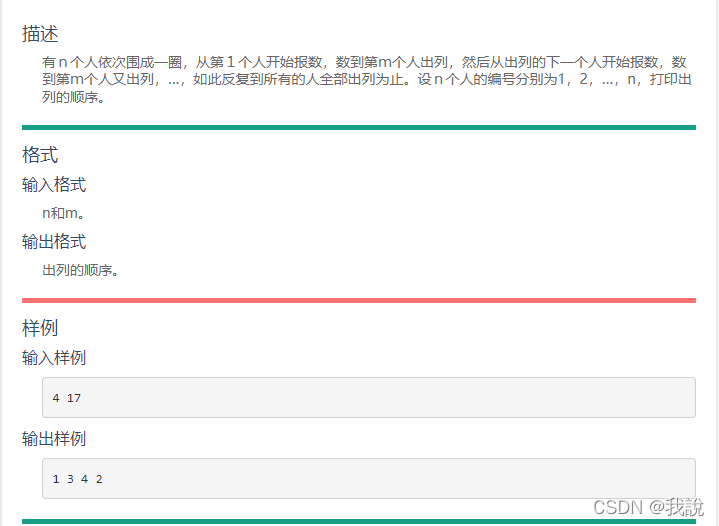C语言围圈报数题目代码实现

围圈报数 题目解题步骤

一.整体逻辑

1.从第１个人开始报数，
2.数到第ｍ个人出列，
3.然后从出列的下一个人开始报数，
4.数到第ｍ个人又出列，…，如此反复到所有的人全部出列为止。

二.逻辑的实现

1.创造一个数组来记录人的信息
2.利用循环依次报数（当只剩下一个人的时候，循环结束（因为不需要报数了，直接输出就可以了））
3.输出报到m的人，并且将他标记起来（表示此人已出列）
4.最后输出剩下的那个人

三.代码的实现

#include
#include
#pragma warning(disable:4996)
int main()
{
int n, m;
scanf("%d %d", &n,& m);
int* arr = (int*)malloc(sizeof(int) * (n+1));  //创造一个数组
for (int i = 0; i <= n; i++)
{
arr[i] = i ;
}
int number = 0;//出列的人数
int k = 0;
int j = 1;
while (number < n - 1)  //当只剩下一个人的时候推出
{
if (arr[j] != 0)//报数
{
k++;
}
if (k == m)
{
printf("%d ", arr[j]);
arr[j] = 0; //出列的人标记起来
number++;
k = 0;
}
j++;
if (j == n+1)        //当最后一个人报完的时候，回到第一个人
j = 1;
}
for (int i = 1; i <= n; i++)
{
if (arr[i] != 0)
printf("%d", arr[i]);  //最后打印出剩下的一个人
}
return 0;
}